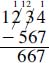NextPrevious

# What does borrow mean in subtraction?

Like “carry” in addition, to “borrow” in subtraction means to take amounts from one number and assign them to the next. In this procedure, the 10 is borrowed from the next higher digit column in order to obtain a positive difference in the nearby column. For example, borrowing is evident in the following operation in the base 10 numbering system (1,234 minus 567, giving the result of 667):When you subtract the same columns, starting in the right hand column, the 4 is too small to subtract from 7; therefore, you need to borrow 10 from the column to the left, boosting the number 4 to 14. The column to the left then loses ten, and 2 is left. Again, the 2 is too small to subtract from 6, so 10 is borrowed from the next column to the left, boosting the number 2 to 12. The last number to the left is dropped down by 10, giving 11; the 5 is subtracted from the 11, resulting in 6 in that column.

Close

This is a web preview of the "The Handy Math Answer Book" app. Many features only work on your mobile device. If you like what you see, we hope you will consider buying. Get the App